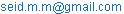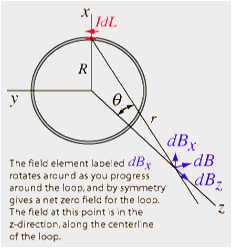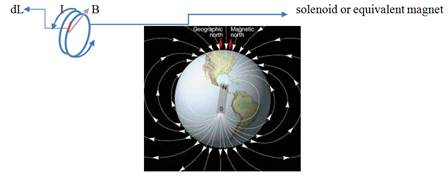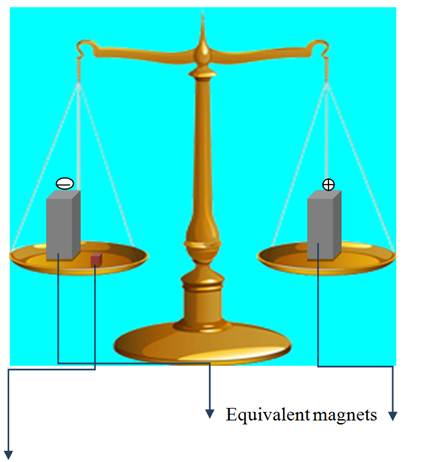International Journal of Science, Technology and Society
Volume 3, Issue 4, July 2015, Pages: 120-123

Electro-Gravitational Effect

Department of Chemical engineering, Sharif University of technology, Tehran, IranSeyedmohammad Mohammadi. Electro-Gravitational Effect. International Journal of Science, Technology and Society. Vol. 3, No. 4, 2015, pp. 120-123. doi: 10.11648/j.ijsts.20150304.14

Abstract: Unity of force fields have been considered by Scientists long time ago. On relation with four fundamental forces the unification of the weak nuclear and electromagnetic forces was formulated and proved by Professor Abduls Salam before. Also significant progress has been made In relation to electrical and strong nuclear forces unity. Following article is clearly proof electro-gravitational field according to the «Electro gravitational effect». According to the theoretical calculations there is a difference weight between weighing a magnet from different pols(without the neglect able magnetic effect of earth) .The difference , which is practically proved in a good approximation , confirms the accuracy of claim.

Keywords: Magnet, Coil, Electromagnetic, Electro-Gravity, Complex Mass, United Field

Contents

1. Introduction

All of the natural forces are originally from four basic forces. Long-range forces of them are

Gravitational and Electromagnetic and the short-ranges are the weak and strong nuclear forces.

According to the current understanding of physics, forces are not transmitted directly between interacting objects, but instead are described by intermediary entities called fields.

Unified field theory(UFT) have been considered by Scientists long time ago 1.The first successful classical unified field theory was developed by James Clerk Maxwell in electromagnetic field . Then Albert Einstein continued subject to the special and general relativities and the other scientists continuous in different ways.

On relation with four fundamental forces, the unification of the weak interaction and electromagnetic forces was formulated by Weinberg, Salam, and Glashow2. They represented that W and Z particles, the intermediate vector bosons, interact in weak nuclear force field. Electromagnetic part is the massless photon and the exchange particles for the weak interaction are the massive W and Z particles. These heavy particles(With masses around 80 and 90 Gev) appeared when the symmetry was spontaneously broken at energy drop below about 80 GeV.

The next step is the inclusion of the strong interaction in what is called grand unification 3,4. Today, one of the major goals of particle physics is to unification of the various fundamental forces in a Grand Unified Theory, which could offer a more elegant understanding of the organization of the universe, is one the major goals of particle physics.

One prediction of the grand unified theories is that the proton is unstable at some level.

Following article clearly proofs electro-gravitational field unification according to the electro gravitational effect.

According to the theoretical calculations there is a difference weight between weighing a magnet from different pols(without the neglectable magnetic effect of earth).The difference , which is practically proved in a good approximation , confirms the accuracy of claim.

Electromagnetic field formulation; separates magnetic flow from electrical flow by the ι, factor5.

Also earth effect on the mentioned coil (or on the equivalent magnet) point out the gravitational flow alike the magnetic flow. Adaptive modulation of synonymous items will unit gravitational and electrical fields in a complex formulation .

2. Calculations

According to the Biot-Savart law; magnetic field on the axis(z direction) of a cylindrical coil, with electrical current, is calculated as following6,7.Fig. 1. magnitude of magnetic field along centerline of a current-carrying loop.

Bz=[μNI R2/2(R2+z2)3/2]»0NIA/2pz3)=[(μ0NI/L)=Bpo][(AL=V)/2pz3] : z>>R

Bz= magnitude of magnetic field of coil center : tesla

μ=[ (μr=relative permeability) (μ0=permeability constant=4p*10-7 tesla)] : tesla

N=turns number : No

I= coil current : amperes

z=axial distance from the center of coil : meters

Bpo =magnitude of magnetic field at pol of coil : tesla

L=coil length : meters

V=coil volume : cubic meters

If z ,direction is same as the earth radius direction then there will be a difference weight between weighing a magnet from different pols. Earth Magnetic effect on weighing, calculated as following, is too small and neglect-able8,9,10:Fig. 2. Earth magnetic field effect on the weight of a solenoid or equivalent magnet.

F=∫02np(v.dq=I.dl)´B

B=local earth magnetic magnitude

n=turn numbers

=> F≈0

The only Explanation and justification for this difference , which unifies gravitational field with electrical field in a complex formulation , is the magneto-gravitational equivalence.

Electro-gravitational unity will be occurred with considering electro-mass equivalence factor (ι.ſ) ; as following :

Fm 1®2= -(G.m1.m2)/d2=[K(m1/ſ)(m2/ſ)]/d2

Fq 1®2 = (K.q1.q2)/d2 = -[G(ſ.q1)(ſ.q2)]/d2

=> ſ =(K/G)0.5=[(9*109Nm2/C2)/(6.67*10-11Nm2/kg2)] 0.5=1.16*1010 kg/C

According to the above equation; gravitational field effect of the Earth on the mentioned coil (or on the equivalent magnet) is as the below :

F={BR=(μNIA/2pR3)=[(μNI/L)=Bpo][(AL=V)/2pR3]}(cM/ſ)

m earth =earth mass=5.972*1024 kg

c=light speed=3*108meters/second

as we expect ; for a magnet with : Bpo0.4 tesla & V=2 *10-5cubic meters we will have :

(|ΔF| : N)(1000/9.81)=2(102BpoVc m earth)/2pſR3=(0.15gr=|Δw|teoritical)≈(0.14gr=|Δw|practical)

3. Experimental Method and Measurement

Digital weighing scales are affected under strong magnet effect. To ensure from results; measuring was carried out in the laboratory of the laboratory Sharif University of Technology by the Pendulum weighing scales . All repeated measurements represent one result (0.14 gr) for weight difference.|Δw|=0.14gr≈0.15gr ; V=20 cm3, B= 4000G

Fig. 3. comparing the weights of tow Equivalent magnets at Weighing in different pols directions.

4. Result

According above items we will have:

1-4-The permanent magnet points out a difference weight between carefully weighing from pols.

2-4-The effect of earth magnetic field on weighing is too small and neglect able.

3-4-The only explanation for this difference; is the magneto-gravitational equivalence.

4-4-Magneto-gravitational fitness; requires to consider the electro-mass equivalence factor (ι.ſ).

5-4-Practical conformity of theoretical forecast confirms the electro-gravitational unity in the complex format. This theory is being completed in an article by «complex gravity» title .

Unmodified shape of the theory , in short form , is as following :

Maxwell equations ( in the anti-commute tensor system ) logically result Electric charge continuity . In a Mutual face tonsorial shape of gravitational equation , which is confirmed with gravitational waves , is resulted from continuity of mass .

Tonsorial compatibility , which is requisite of mass and charge continuity , ceases an electro- gravitational modulation of them in complex time-space in the anti-commute tensor formation.

As for to the self-identity between mass and charge and according to the Interaction between them ; electro-mass equivalence factor (ι.ſ) is calculated as following :

Fm 1®2= -(G.m1.m2)/d2=[K(m1/ſ)(m2/ſ)]/d2

Fq 1®2 = (K.q1.q2)/d2 = -[G(ſ.q1)(ſ.q2)]/d2

=> ſ =(K/G)0.5=[(9*109Nm2/C2)/(6.67*10-11Nm2/kg2)] 0.5=1.16*1010 kg/C

As for the mentioned items ; Interaction between particles will be as following :

{[Fi=D(MiVi)/Dt]=[G.MiMj/(L=|Li,j|)2][UFj(Uj=Vj/c)(Ui=Vi/c)=UFi] <=>

[Fj=D(MjVj)/Dt]=[G.MjMi/(L=|Lj,i|)2][UFi(Ui=Vi/c)(Uj=Vj/c)=UFj]} :

Lim UFi=(Ui,j=Li,j/L) , LimUFj=(Uj,i=Lj,i/L)

V1 ,V2 →cV               V1 ,V2 →c

=> [(UFj=DVi/Dt)(Uj=Vj/c)]=[(UFi=DVj/Dt)(Ui=Vi/c)]       =>

Vi=c{coshiiat+ti,0)[(cosθi)Ћ-(sinθi)k]+ί(sinhτi)[cos(θiibq+qi,0)i+(sinθi)j]}

Vj=c{coshjjat+tj,0)[(cosθj)Ћ-(sinθj)k]+ί(sinhτj)[cos(θjjbq+qj,0)i+(sinθj)j]}

: Гi=∫[G.Mj/(c.L2)]dt , Гj=∫[G.Mi/(cL2)]dt ; at2bq2=1

compatible solution of above equations results the general and widespread formation of gravity .

In the simple, static start, we have:

Fi=[(G.MiMj/L2)=(c.A)MiMj] [(sinhτi)Ћ+ί(coshτi)i] =

(G/L2)[(mimj-ſ2qiqj)+ί.ſ(miqj+mjqi)]{[sinh(A.mj)cos(A.ſ.qj)+ί.cosh(A.mj)sin(A.ſ.qj)]Ћ

+ί[cosh(A.mj)cos(A.ſ.qj)+ί.sinh(A.mj)sin(A.ſ.qj)]i}=(G/L2)[(mimj-ſ2qiqj)+ί.ſ(miqj+mjqi)]

cos(A.ſ.qj)[sinh(A.mj)Ћ+ί.cosh(A.mj)i]+ί.sin(A.ſ.qj)[cosh(A.mj) Ћ+ί.sinh(A.mj)i]

≈(G/L2)[(mjmi-ſ 2qjqi)i

Fj=[(G.MiMj/L2)=(c.A)MiMj][(sinhτj)Ћ+ί(coshτj)i] =

(G/L2)[(mjmi-ſ2qjqi)+ί.ſ(mjqi+miqj)]{[sinh(A.mi)cos(A.ſ.qi)+ί.cosh(A.mi)sin(A.ſ.qi)]Ћ

-ί[cosh(A.mi)cos(A.ſ.qi)+ί.sinh(A.mi)sin(A.ſ.qi)]i}=(G/L2)[(mimj-ſ2qiqj)+ί.ſ(miqj+mjqi)]

cos(A.ſ.qi)[sinh(A.mi)Ћ-ίcosh(A.mi)i]+ί.sin(A.ſ.qi)[cosh(A.mi)Ћ-ί.sinh(A.mi)i]

≈-(G/L2)[(mjmi-ſ 2qjqi)i

References

1. en.wikipedia.org/wiki/Unified_field_theory.
2. http://hyperphysics.phy-astr.gsu.edu/hbase/forces/unify.html.
4. http://hyperphysics.phy-astr.gsu.edu/hbase/forces/unify.html#c5.
5. www.electronspin.org/14.htm.
6. http://ptsg.eecs.berkeley.edu/~helens/edu/sol_page/solenoid.html.
7. http://icecube.wisc.edu/~karle/courses/phys202/202lecture13.pdf.
9. http://ocw.mit.edu/courses/physics/8-02-physics-ii-electricity-and-magnetism-spring-2007/class-activities/ch9sourc_b_field.pdf.
10. http://seismo.berkeley.edu/~rallen/eps122/lectures/L05.pdf.

 Contents 1. 2. 3. 4.
Article ToolsAbstractPDF(582K)